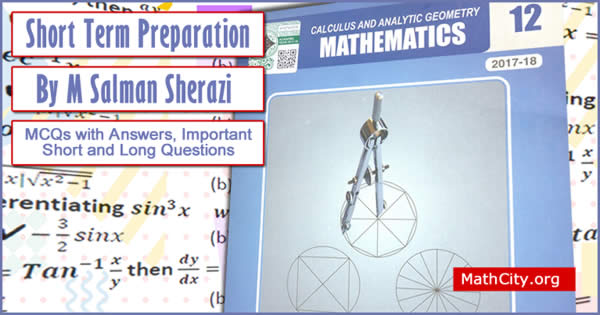# Short Term Preparation FSc 2This document contains all the important MCQs, Short Questions and Long Questions of Mathematics HSSC-II (FSc Part 2) from the Calculus and Analytic Geometry, MATHEMATICS 12. It has been done to help the students and teachers at no cost by M Salman Sherazi. This work (pdf) is licensed under a Creative Commons Attribution-NonCommercial-NoDerivatives 4.0. It has been done to help the students and teachers at no cost by M Salman Sherazi.

• Important MCQs with answers and short questions are written for each exercise of the book.
• For some exercise long questions are also given.

Sample:

1. The notation $y=f(x)$ was invented by
1. Libnitz
2. Euler
3. Newton
4. Lagrange
2. If $f(x)=\frac{x}{x^2-4}$, then domain of $f$ is
1. $\mathbb{R}$
2. $\mathbb{R}-\{0\}$
3. $\mathbb{R}-{\pm 2}$
4. $\mathbb{Q}$
• fsc-part2-ptb/short-term-preparation-salman-sherazi